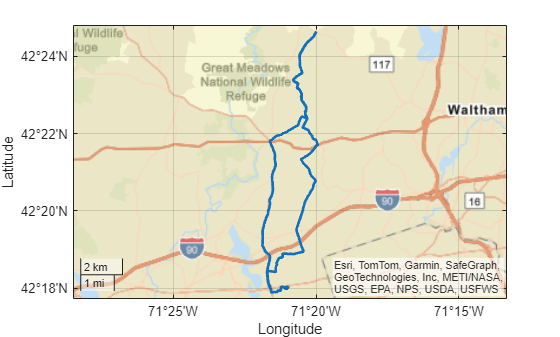# geolineshape

Line shape in geographic coordinates

Since R2021b

## Description

A `geolineshape` object represents a line or multiline in geographic coordinates. A multiline is an individual line shape that contains a set of separate lines.

To represent a line or multiline in planar coordinates, use a `maplineshape` object instead.

## Creation

To create `geolineshape` objects, either:

• Import line data in geographic coordinates as a geospatial table using the `readgeotable` function, and then query the `Shape` variable of the table.

• Use the `geolineshape` function (described here).

### Syntax

``shape = geolineshape(lat,lon)``

### Description

example

````shape = geolineshape(lat,lon)` creates a `geolineshape` object or array of `geolineshape` objects with vertices at the specified latitude and longitude coordinates. The sizes of `lat`, `lon`, and the `geolineshape` object array `shape` match.```

### Input Arguments

expand all

Latitude coordinates, specified as a numeric vector or a cell array of numeric vectors.

• Create a line by specifying a vector, such as ```[65 62 53 66]```.

• Create a multiline by specifying a vector and including line breaks as `NaN` values, such as ```[55 34 18 NaN 14 19 42 26]```.

• Create an array of lines and multilines by specifying a cell array of vectors, such as `{[55 34 18],[14 19 NaN 42 26 31]}`.

The `NaN` values in `lat` must correspond to the `NaN` values in `lon`.

The size of `lat` must match the size of `lon`. For cell arrays, the size of the vector in each cell of `lat` must match the size of the vector in the corresponding cell of `lon`.

Data Types: `double` | `cell`

Longitude coordinates, specified as a numeric vector or a cell array of numeric vectors.

• Create a line by specifying a vector, such as ```[4 59 121 98]```.

• Create a multiline by specifying a vector and including line breaks as `NaN` values, such as ```[78 56 63 NaN 83 106 104 126]```.

• Create an array of lines and multilines by specifying a cell array of vectors, such as ```{[78 56 63],[83 106 NaN 104 126 131]}```.

The `NaN` values in `lat` must correspond to the `NaN` values in `lon`.

The size of `lat` must match the size of `lon`. For cell arrays, the size of the vector in each cell of `lat` must match the size of the vector in the corresponding cell of `lon`.

Data Types: `double` | `cell`

## Properties

expand all

Number of line parts, returned as an array of nonnegative integers.

For a `geolineshape` scalar, the value of `NumParts` is `1` when the `geolineshape` object represents a single line and more than `1` when the object represents a multiline.

For a `geolineshape` array, the size of `NumParts` matches the size of the array.

Data Types: `double`

Geometric type, returned as `"line"`.

Data Types: `string`

Coordinate system type, returned as `"geographic"`.

Data Types: `string`

Geographic coordinate reference system (CRS), specified as a `geocrs` object. A geographic CRS consists of a datum (including its ellipsoid), prime meridian, and angular unit of measurement.

## Object Functions

 `geoplot` Plot points, lines, and polygons on map `geoclip` Clip geographic shape to latitude-longitude limits `ismultipoint` Determine which array elements are multipoint shapes

## Examples

collapse all

Import the `tracks` layer of a GPX file containing routes in Massachusetts as a geospatial table. The `tracks` layer represents the routes using lines. Get information about the line in the second track by querying the `Shape` variable of the table.

```GT = readgeotable("sample_tracks.gpx",Layer="tracks"); GT.Shape(2)```
```ans = geolineshape with properties: NumParts: 5 Geometry: "line" CoordinateSystemType: "geographic" GeographicCRS: [1×1 geocrs] ```

Create a subtable that contains the second track. Display the second track on a road map.

```GT2 = GT(2,:); geoplot(GT2,LineWidth=2) geobasemap streets```Create an individual line as a `geolineshape` scalar. Specify the geographic CRS as the World Geodetic System of 1984, which has the EPSG code `4326`.

```lat = [65 62 53 66]; lon = [4 59 121 98]; lineshp = geolineshape(lat,lon); g = geocrs(4326); lineshp.GeographicCRS = g```
```lineshp = geolineshape with properties: NumParts: 1 Geometry: "line" CoordinateSystemType: "geographic" GeographicCRS: [1x1 geocrs] ```

Create a multiline as a `geolineshape` scalar.

```lat = [55 34 18 NaN 14 19 42 26]; lon = [78 56 63 NaN 83 106 104 126]; multiline = geolineshape(lat,lon); multiline.GeographicCRS = g```
```multiline = geolineshape with properties: NumParts: 2 Geometry: "line" CoordinateSystemType: "geographic" GeographicCRS: [1x1 geocrs] ```

Create one individual line and one multiline as a 1-by-2 `geolineshape` array.

```lat = {[55 34 18],[14 19 NaN 42 26 37]}; lon = {[78 56 63],[83 106 NaN 104 126 113]}; lineMultiline = geolineshape(lat,lon); lineMultiline.GeographicCRS = g```
```lineMultiline=1×2 object 1x2 geolineshape array with properties: NumParts: [1 2] Geometry: "line" CoordinateSystemType: "geographic" GeographicCRS: [1x1 geocrs] ```

## Version History

Introduced in R2021b